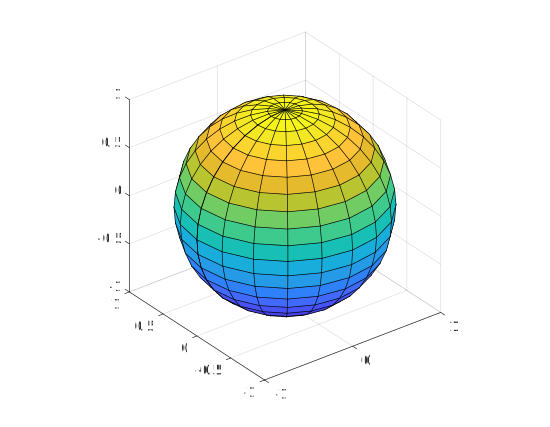Question #5835

# How to draw a sphere in Matlab ?

Merged questions

In Matlab, there is no function to draw a sphere. Therefore, the ﻿`sphere` keyword returns the—ready-to-draw—coordinates of a sphere. The coordinates can be plotted with the ﻿`surf` function:

``````[X,Y,Z] = sphere;
surf(X,Y,Z);
axis square equal;``````To specify the center and the radius of the sphere, use the following code :

``````[X,Y,Z] = sphere;